Name:    2nd Grade Measurement and Data- Measure and estimate lengths in standard units, Standard 4, Pre Test 1 Measure to determine how much longer one object is than another, expressing the length difference in terms of a standard length unit.

Multiple Choice
Identify the choice that best completes the statement or answers the question.

1.How much longer is the line than the hexagon?
 a. 4 yards c. 4 centimeters b. 3 inches d. 4 inches

2.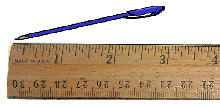How much longer is the pen than the eraser?
 a. 4 centimeters c. 3 inches b. 1 inch d. 1 foot

3.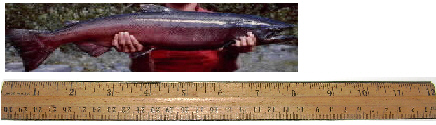How much longer is the salmon than the trout?
 a. 3 inches c. 3 feet b. 1 inch d. 3 centimeters

4.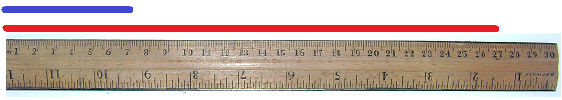How much longer is the red line than the blue line?
 a. 20 inches c. 20 centimeters b. 1 foot d. 30 centimeters

5.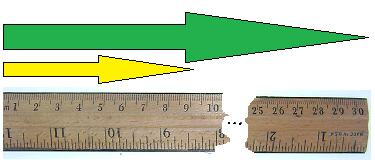How much longer is the green arrow than the yellow arrow?
 a. 20 inches c. 10 centimeters b. 1yard d. 21 centimeters

6.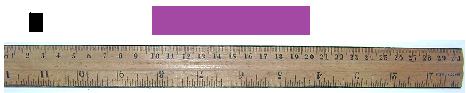How much longer is the purple line than the black line?
 a. 19 centimeters c. 20 inches b. 9 centimeters d. 1/2 foot

7.How much longer is the bomber model on the bottom than the jet model on the top?
 a. 10 centimeters c. 10 inches b. 2 feet d. 1 foot